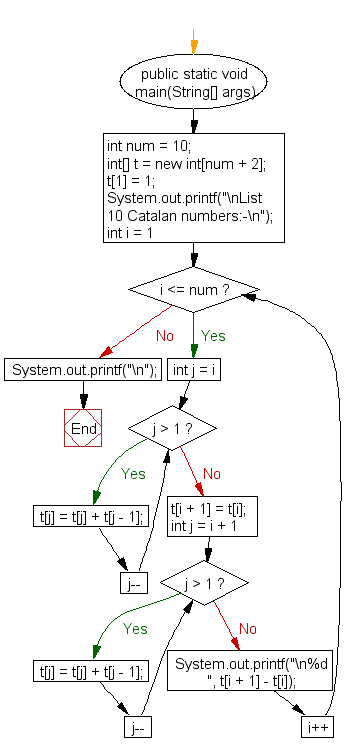﻿ Java exercises: Print out the first 10 Catalan numbers by extracting them from Pascal's triangle - w3resource# Java Exercises: Print out the first 10 Catalan numbers by extracting them from Pascal's triangle

## Java Numbers: Exercise-8 with Solution

Write a Java program to print out the first 10 Catalan numbers by extracting them from Pascal's triangle.

In combinatorial mathematics, the Catalan numbers form a sequence of natural numbers that occur in various counting problems, often involving recursively-defined objects. They are named after the Belgian mathematician Eugène Charles Catalan.
The first Catalan numbers for n = 0, 1, 2, 3, … are 1, 1, 2, 5, 14, 42, 132, 429, 1430, 4862, 16796, 58786, 208012, 742900, 2674440, 9694845, 35357670, 129644790, 477638700, 1767263190, 6564120420, 24466267020, 91482563640, 343059613650, 1289904147324, 4861946401452,

Sample Solution:

Java Code:

``````import java.util.Scanner;
public class Example8 {
public static void main(String[] args) {
int num = 10;
int[] t = new int[num + 2];
t = 1;
System.out.printf("\nList 10 Catalan numbers:-\n");
for (int i = 1; i <= num; i++) {

for (int j = i; j > 1; j--)
t[j] = t[j] + t[j - 1];

t[i + 1] = t[i];

for (int j = i + 1; j > 1; j--)
t[j] = t[j] + t[j - 1];
System.out.printf("\n%d ", t[i + 1] - t[i]);
}
System.out.printf("\n");
}
}
```
```

Sample Output:

```List 10 Catalan numbers:-
1
2
5
14
42
132
429
1430
4862
16796
```

Flowchart:Java Code Editor:

What is the difficulty level of this exercise?

﻿

## Java: Tips of the Day

Array vs ArrayLists:

The main difference between these two is that an Array is of fixed size so once you have created an Array you cannot change it but the ArrayList is not of fixed size. You can create instances of ArrayLists without specifying its size. So if you create such instances of an ArrayList without specifying its size Java will create an instance of an ArrayList of default size.

Once an ArrayList is full it re-sizes itself. In fact, an ArrayList is internally supported by an array. So when an ArrayList is resized it will slow down its performance a bit as the contents of the old Array must be copied to a new Array.

At the same time, it's compulsory to specify the size of an Array directly or indirectly while creating it. And also Arrays can store both primitives and objects while ArrayLists only can store objects.

Ref: https://bit.ly/3o8L2KH